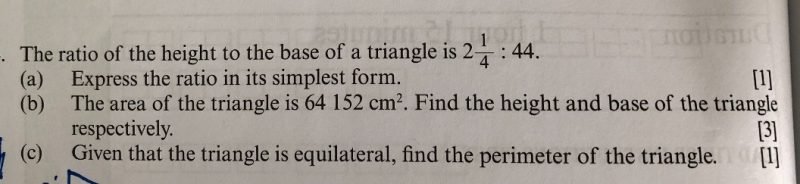# Questiona) h  :  b  ->  9/4  :   44   -> 9  :  176

b)  Area of triangle = 1/2  x   b  x   h -> 1/2 x 9u x 176u -> 64152

u2 -> (64152 x 2) / (9 x 176) = 81

1u  -> 9

Height of triangle -> 9u -> 9 x 9 = 81 cm

Base of triangle -> 176u  -> 176 x 9 = 1584 cm

c) perimeter of equilateral triangle = 3 x base = 3 x 1584 = 4752 cm

0 Replies 1 Like